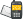It is currently 24 Nov 2020, 10:10### GMAT Club Daily Prep

#### Thank you for using the timer - this advanced tool can estimate your performance and suggest more practice questions. We have subscribed you to Daily Prep Questions via email.

Customized
for You

we will pick new questions that match your level based on your Timer History

Track

every week, we’ll send you an estimated GMAT score based on your performance

Practice
Pays

we will pick new questions that match your level based on your Timer History

#### Not interested in getting valuable practice questions and articles delivered to your email? No problem, unsubscribe here.# If x^2 + 4x + 3 is odd, then which one of the following couQuestion banks Downloads My Bookmarks Reviews Important topics
Author Message
TAGS:
FounderJoined: 18 Apr 2015
Posts: 13889
GRE 1: Q160 V160Followers: 313

Kudos [?]: 3676 , given: 12909

If x^2 + 4x + 3 is odd, then which one of the following cou [#permalink]
Expert's post00:00

Question Stats:82% (00:38) correct17% (00:36) wrongbased on 23 sessions
If $$x ^2 + 4x + 3$$ is odd, then which one of the following could be the value of x ?

(A) 3
(B) 5
(C) 9
(D) 13
(E) 16
[Reveal] Spoiler: OA

_________________InternJoined: 03 Mar 2015
Posts: 14
Followers: 0

Kudos [?]: 17  , given: 2

Re: If x^2 + 4x + 3 is odd, then which one of the following cou [#permalink]
1
KUDOS
It is good to note that
Odd + Odd = Even
Even + Even = Even
Odd + Even = Odd

&
Odd * Odd = Odd
Even * Even = Even
Even * Odd = Even

Now coming to the question:
It states x^2 + 4x + 3 is ODD

We know that whatever be the value of x, 4x will always be even (Even * Odd (or Even) = Even)
We also know that 3 is Odd

Therfore we can rewrite the expression in the question: x^2 + 4x + 3 is Odd as
x^2 + Even + Odd is Odd

We know (Even + Odd) is Odd

Therefore,
x^2 + Odd is Odd

For the above to be true,
x^2 has to be even and this will happen only when x is EVEN.

The only even number in the answer choice is 16.

_________________

Press Kudos if you find the post usefulRegards
Ambarish
GMAT/GRE/SAT trainer
My Reviews: https://www.urbanpro.com/bangalore/amba ... ws/7229395
Message/Whatsapp: +91-9686497804

ManagerJoined: 26 Jan 2018
Posts: 189
GRE 1: Q165 V156Followers: 1

Kudos [?]: 136 , given: 3

Re: If x^2 + 4x + 3 is odd, then which one of the following cou [#permalink]
On solving for z, one value we get for x is -3.

Square of x in that case would be 9. Can this not be the answer?InternJoined: 03 Mar 2015
Posts: 14
Followers: 0

Kudos [?]: 17  , given: 2

Re: If x^2 + 4x + 3 is odd, then which one of the following cou [#permalink]
1
KUDOS
Hey Mohan,

Did you assume x^2 + 4x + 3 = 0 ??

Note that the question did not mention that x^2+4x+3 is zero. In fact it says, it is some “ odd number”

Hope this helpsPosted from my mobile device_________________

Press Kudos if you find the post usefulRegards
Ambarish
GMAT/GRE/SAT trainer
My Reviews: https://www.urbanpro.com/bangalore/amba ... ws/7229395
Message/Whatsapp: +91-9686497804ManagerJoined: 06 Jun 2018
Posts: 94
Followers: 2

Kudos [?]: 80  , given: 0

Re: If x^2 + 4x + 3 is odd, then which one of the following cou [#permalink]
1
KUDOS
Carcass wrote:
If $$x ^2 + 4x + 3$$ is odd, then which one of the following could be the value of x ?

(A) 3
(B) 5
(C) 9
(D) 13
(E) 16

Plugging in the smaller values Such as 1 , 2 etc to watch the pattern.

x = 1 ( odd )

(1)^2 + 4*1 + 3 = 8 ( even ) . It means we have to pick up the even value for x. Let's try:

(2)^2 + 4*2 + 3 = 15 ( odd ) .That fits.

Only even number is 16.Re: If x^2 + 4x + 3 is odd, then which one of the following cou   [#permalink] 17 Jul 2018, 12:33
Display posts from previous: Sort by

# If x^2 + 4x + 3 is odd, then which one of the following couQuestion banks Downloads My Bookmarks Reviews Important topicsPowered by phpBB © phpBB Group Kindly note that the GRE® test is a registered trademark of the Educational Testing Service®, and this site has neither been reviewed nor endorsed by ETS®.